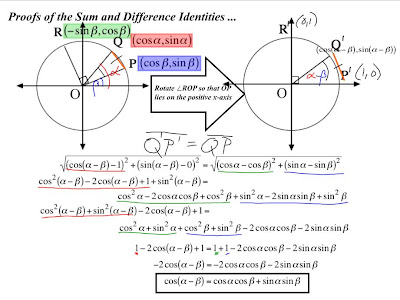## Monday, March 24, 2008

### Proofs; The Sum and Difference Identities.

Alright, lets get this show on the road as I'm now the replacement scribe for today. First things first.

PAUL IS THE SCRIBE FOR TOMORROW (March 25th, 2008)

Anyways now that thats dealt with lets begin.

We started class off with a quiz, which for many of us (or at least I think it was many of us) had a bit of trouble getting into. One of the main points to remember in this little intro portion from the quiz was to look at difference of squares, and look out for one of the factors of them. Many of the questions were solved by algebraically massaging the expression using a difference of squares (where applicable.) Anyways the answers to the quiz(and in most cases a step by step guide of how to get to those answers) can be found;

Here

Things to Remember
-Look for a difference of squares, or a factor of a difference of squares
-Remember that you dont need to memorize the corollaries for the trig identities, but rather, derive them yourself when you need them from the Pythagorean theorem. Sine^2 + Cosine ^ 2 = 1.

*Note* In case the images are to small, they're all on the slides, so simply jet on over to the link above and hunt em down :)

So with that little opening bit of the lesson out of the way, lets continue with the main content of this scribe post, The proofs of the Sum and Difference Identities.

If you remember from the previous class on Thursday, we were right on the precipice of uncovering the, "clever idea" behind these identities. If you recall, we worked with the angles ROP, and QOP. Now assuming the angle was in a unit circle, we were able to find the coordinates of points Q, P, and R in terms of Sine and Cosine. After that we implemented the first portion of our clever idea, a rigid transformation of the overall angle, which put it on the x and y axis perfectly. The finding of the angles all lie in the fact that angle QOP, is equivalent to angle alpha subtract angle Beta. Thus when the whole thing is rotated, the angle QOP on the x axis may be described as Alpha - Beta.Now that our recap is completed lets get into the new stuff! By looking at the above you may see an orange line. This represents the distance between Q and P. This here, is the bread and butter of the clever idea for today. So with the rigid transformation from the first to second diagram, the distance from Q to P must have remained the same (hence rigid transformation.) It is from this point the real magic can be worked. Next we used the Distance formula √[(X2-X1)^2 + (Y2-Y1)^2] (which is the Pythagorean theorem by the way.) and plugged in the values we found before. Thus we got everything seen on the following slide.Once the Sine and Cosine values have been plugged in you begin to simplify it all down until you have the cosine difference identity. Nice eh? Now because that only covered the difference identity, we have to find the sum identity. To advance here however, we must go back, to Odd and Even functions. If your memory serves you correctly you should remember that Cosine is an Even function and Tangent, and Sine are both Even functions, that is to say that Cosine (x) = Cosine (-x), Tan(-x) = -Tangent(x), and Sine(-x) = -Sine(x). So keeping all that in mind, and remembering we have already proven one identity, we can now prove the cosine sum identity As follows

Cos(α+β) = Cos (α-[-β]) <---------- Clever Idea!
|
Cos (α) Cos (-β) + Sin (α) Sin (-β)

|
Cos (α) Cos (β) + Sin (α) Sin (β) *
Cos(
α+β) = | Cos (α) Cos(β) + Sin (α) Sin (β)By substituting in the even or odd identity for Sine and Cosine we were able to come up with the proof for the Sum identities.

Now I think that wraps up just about everything we did in this class. Today.
Remember that homework for today is to (using the above) come up with the sum and difference proofs for Sine. To do this use what we learned today, on R'Q' = RQ rather then Q'P' = QP. Also we have Exercise the Next, which is known more specifically as Exercise 17.

So ja, thats it. Hopefully it made sense. In case you missed it up top, paul is scribe for tomorrow. Now I must go build a catapult for physics! -_-;

#### 1 comment:

m@rk said...

A very well detailed scribe post. This scribe post helped me remember the concept behind this specific identity. I remember the fist time that i learned this identity, i found it really dry and boring. Now, i just need to put this found knowledge in the upcoming test. Keep up the good work!

-m@rk